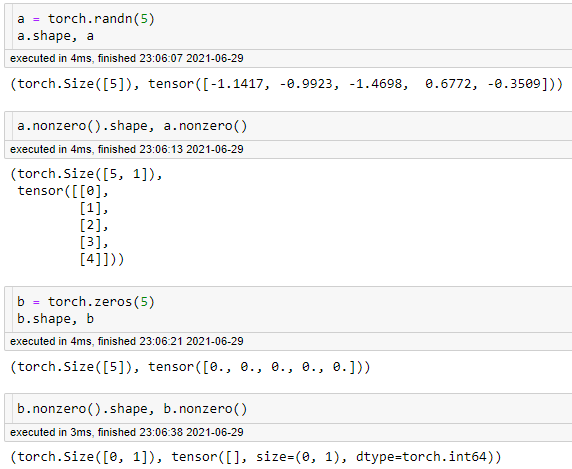# Check if at least one element in tensor is nonzero

I want to check if a tensor contains nonzero elements. It does not matter to me how many nonzero elements there are. I currently do the following which is very inefficient for large tensors:

`T.abs().sum().item() == 0`

Is there a better way to do this in PyTorch? Like a function that stops iterating through the tensor as soon as it finds the first nonzero element?

Not too sure on that, but I do believe that this:

`T.abs().sum().item() == 0`

will be much faster than

a function that stops iterating through the tensor as soon as it finds the first nonzero element?

as loops/iterators are very very slowAlso this might be of interest: `.nonzero()`You can check `some_tensor.nonzero()` and check for empty results (i.e. `tensor([])`

1 Like

tensorName.type(t.uint8).any()

I guess `tensor_name.any()` should work fine. Why the `type()` call?